### Input Data Sets

The BOUNDARY= data set option is required, and if neither the DATA= nor the PARMS= data set option is specified, the procedure derives statistics such as Type I and Type II error probabilities from the BOUNDARY= data set. The resulting boundaries are displayed with the scale specified in the BOUNDARYSCALE= option.

#### BOUNDARY= Data Set

The BOUNDARY= data set provides the boundary information for the sequential test. At stage 1, the data set is usually created with an ODS OUTPUT statement from the Boundary Information table created by the SEQDESIGN procedure. At each subsequent stage, the data set is usually created with an ODS OUTPUT statement from the Test Information table that was created by the SEQTEST procedure at the previous stage. See the section Getting Started: SEQTEST Procedure for an illustration of the BOUNDARY= data set option.

The BOUNDARY= data set contains the following variables:

• _Scale_, the boundary scale, with the value MLE for the maximum likelihood estimate, STDZ for the standardized Z, SCORE for the score statistic, or PVALUE for the nominal p-value. Note that for a two-sided design, the nominal p-value is the one-sided fixed-sample p-value under the null hypothesis with a lower alternative hypothesis.

• _Stop_, the stopping criterion, with the value REJECT for rejecting the null hypothesis, ACCEPT for accepting, or BOTH for both rejecting and accepting• _ALT_, the type of alternative hypothesis, with the value UPPER for an upper alternative, LOWER for a lower alternative, or TWOSIDED for a two-sided alternative

• _Stage_, the stage number

• the boundary variables, a subset of Bound_LA for lowerboundary, Bound_LB for lowerboundary, Bound_UB for upperboundary, and Bound_UA for upperboundary

• AltRef_L, the lower alternative reference, if ALT=LOWER or ALT=TWOSIDED

• AltRef_U, the upper alternative reference, if ALT=UPPER or ALT=TWOSIDED

• _InfoProp_, the information proportion at each stage

Optionally, the BOUNDARY= data set also contains the following variables:

• _Info_, the information level at each stage

• NObs, the required number of observations for nonsurvival data at each stage

• Events, the required number of events for survival data at each stage

• Parameter, the variable specified in the DATA(TESTVAR=) or PARMS(TESTVAR=) option

• Estimate, the parameter estimate

If the BOUNDARY= data set contains the variable Parameter for the test variable that is specified in the TESTVAR= option, and the variable Estimate for the test statistics, then these test statistics are also displayed in the output test information table and output test plot.

#### DATA= Data Set

The DATA= data set provides the test variable information for the current stage of the trial. Such data sets are usually created with an ODS OUTPUT statement by using a procedure such as PROC MEANS. See Testing a Binomial Proportion for an illustration of the DATA= data set option.

The DATA= data set includes the following variables:

• _Stage_, the stage number

• _Scale_, the scale for the test statistic, with the value MLE for the maximum likelihood estimate, STDZ for the standardized Z, SCORE for the score statistic, or PVALUE for the nominal p-value

• _Info_, the information level

• NObs, the number of observations for nonsurvival data at each stage

• Events, the number of events for survival data at each stage

• test variable, specified in the TESTVAR= option, contains the test variable value in the scale specified in the _Scale_ variable

With the specified DATA= data set, PROC SEQTEST derives boundary values from the information levels in the _Info_ variable. If the data set does not include the _Info_ variable, then the information levels are derived from the NObs or Events variable in the DATA= data set if that variable is also in the input BOUNDARY= data set. That is, the information level at stage k is computed as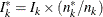, where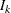and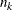are the information level and sample size, respectively, at stage k in the BOUNDARY= data set and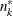is the sample size at stage k in the DATA= data set. Otherwise, the information levels from the BOUNDARY= data set are used.

If the TESTVAR= option is specified, the DATA= data set must also include the test variable for the test statistic and _Scale_ variable for the corresponding scale. Note that for a two-sided design, the nominal p-value is the one-sided fixed-sample p-value under the null hypothesis with a lower alternative hypothesis.

#### PARMS= Data Set

The PARMS= data set provides a parameter estimate and associated standard error for the current stage of the trial. Such data sets are usually created with an ODS OUTPUT statement by using procedures such as the GENMOD, GLM, LOGISTIC, and REG procedures. See the section Getting Started: SEQTEST Procedure for an illustration of the PARMS= data set option.

The PARMS= data set includes the following variables:

• _Stage_, the stage number

• _Scale_, the scale for the test statistic, with the value MLE for the maximum likelihood estimate, STDZ for the standardized Z, SCORE for the score statistic, or PVALUE for the nominal p-value

• Parameter, Effect, Variable, or Parm, which contains the variable specified in the TESTVAR= option

• Estimate, the parameter estimate

• StdErr, standard error of the parameter estimate

• NObs, the number of observations for nonsurvival data at each stage

• Events, the number of events for survival data at each stage

With the specified PARMS= data set, the information level is derived from the StdErr variable. For a score statistic, the information levelis the variance of the statistic,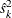, where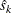is the standard error in the StdErr variable. Otherwise, the information level is the inverse of the variance of the statistic,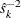. If the data set does not include the StdErr variable, the information levels derived from the BOUNDARY= data set are used.

If the data set does not include the StdErr variable, then the information levels are derived from the NObs or Events variable in the PARMS= data set if that variable is also in the input BOUNDARY= data set. That is, the information level at stage k is computed as, whereandare the information level and sample size, respectively, at stage k in the BOUNDARY= data set andis the sample size at stage k in the PARMS= data set. Otherwise, the information levels from the BOUNDARY= data set are used.

If the TESTVAR= option is specified, the PARMS= data set also includes the variable Parameter, Effect, Variable, or Parm for the test variable, Estimate for the test statistic, and _Scale_ variable for the corresponding scale. Note that for a two-sided design, the nominal p-value is the one-sided fixed-sample p-value under the null hypothesis with a lower alternative hypothesis.